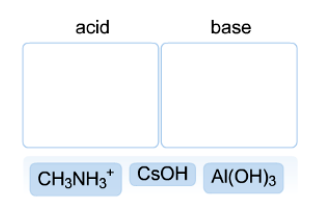# Problem: Using the Arrhenius theory, classify the following as either an acid or a base, by placing the compound in the correct bin.

###### FREE Expert Solution
84% (212 ratings)
###### Problem Details

Using the Arrhenius theory, classify the following as either an acid or a base, by placing the compound in the correct bin.Frequently Asked Questions

What scientific concept do you need to know in order to solve this problem?

Our tutors have indicated that to solve this problem you will need to apply the Arrhenius Acid and Base concept. You can view video lessons to learn Arrhenius Acid and Base. Or if you need more Arrhenius Acid and Base practice, you can also practice Arrhenius Acid and Base practice problems.

What is the difficulty of this problem?

Our tutors rated the difficulty ofUsing the Arrhenius theory, classify the following as either...as medium difficulty.

How long does this problem take to solve?

Our expert Chemistry tutor, Dasha took 2 minutes and 53 seconds to solve this problem. You can follow their steps in the video explanation above.

What professor is this problem relevant for?

Based on our data, we think this problem is relevant for Professor Robinson's class at UW-SEATTLE.